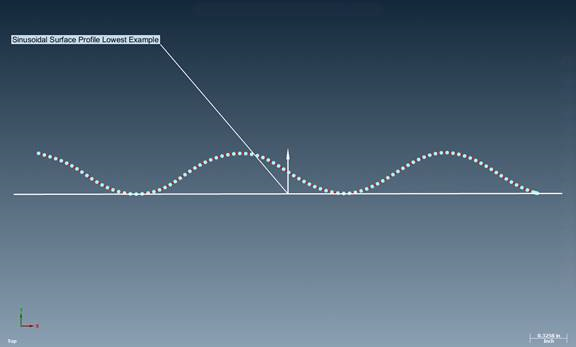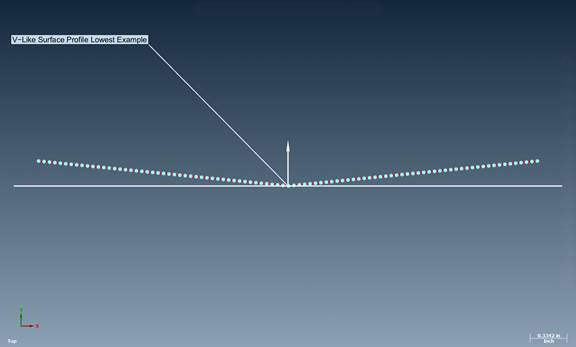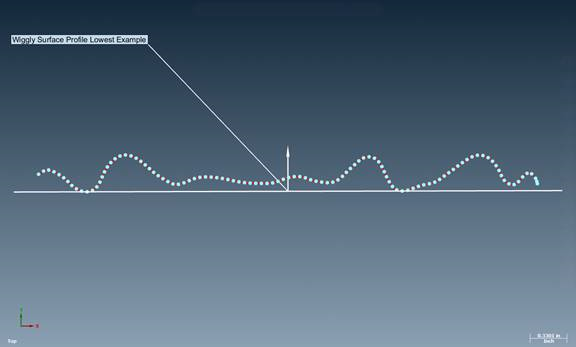# Plane Fitting

### Case:

Regarding the fitting algorithm, can you give me any more information on how each of the Minimum Zone fits work? We need a fit that simulates a surface plate alignment by using only the three highest points measured.

### Description:

Verisurf follows ASME Y14.5.1-2019 Mathematical Definition of Dimensioning and Tolerancing Principles.

Specifically, we use Section-2.2.1 for Least Squares (Gaussin) and Section B-2.2.2 Minimum Zone (Min-Max/Chebyshev). The Constraints, Highest and Lowest, are per Section B-2.3.2. Section B-2.6 recommends using the Least Square Constrained method for most datums at RMB.

Here are a few references I have simplified from the ASME Y14.5.1-2019 specification to help us understand and answer this question.

Section B-2.2.1 Least Squares, The simplest definition for a single simulator is that of least squares (also called Gaussian). The chosen simulator is the surfaces that minimizes the sum (integral) of squared distances between S and the datum feature. The least squares simulator’s greatest strength is its repeatability across parts: similarly manufactured parts will almost always have highly similar datum simulators.

Section B-2.2.2 Min-Max, Another definition for a single simulator is known as min-max; it is also known as Chebyshev, minimax, and minimum zone. The min-max simulator surfaces is the surface that minimizes the maximum absolute distance between S and the datum feature. Per this definition, the worst deviation (also known as residual) is as small as possible. This property is why min- max fitting is used in many tolerance calculations (cylindricity, flatness, perpendicularity, etc.).

Section B-2.3.2 Constrained Norm-Minimizing Strategies, Another way to define an external simulator is to add constraints to the optimization problem. Any norm can be minimized, but a constraint is added such that the simulator is required to minimize the norm while being external to the material. In other words, minimize the integrated squared distances, while requiring all the signed distances to be non-negative.

By contrast, the constrained min-max simulator is the same as an unconstrained min-max simulator that has been progressed to be external to the material. Since this definition minimizes the worst-case distance, it is sensitive to outliers. This simulator has excellent externality, moderate repeatability across similar parts, and poor contact and plausibility.

The constrained least squares simulator (constrained L2) behaves remarkably well. In cases where there are clear points of contact that a metrology engineer would choose, the constrained least squares simulator chooses them. In cases where the part "rocks,” the constrained least squares simulator chooses an equalized solution that a metrology engineer might have chosen. In summary of the detailed comparison below, the constrained least squares simulator has excellent externality and contact. It has good plausibility, and good repeatability across parts.

Section B-2.6 Summary and Recommendations, for most datums referenced at RMB, it is recommended to use the constrained least squares (constrained L2) simulator. In cases of planar datums referenced at RMB, the constrained least squares simulator (constrained L2) applied to the external envelope is recommended.

Below are screenshots of the 3 examples using the Minimum Zone Lowest Method in Verisurf. The white arrow in the middle represents the normal or vector of the plane, and the white line below each dataset represents the location or position of the plane.

The reason for the Minimum Zone Lowest, as opposed to the Minimum Zone Highest, is due to the vector of the plane. Notice the plane vector in the examples below are all pointing up. In these scenarios, the plane method would be set to the lowest to represent how the primary datum feature would be set on a surface plate.We hope you found this information helpful.

If there are any further questions, please call Verisurf Support at 1-888-713-7201